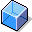Costing SheetDefinition

The costing sheet combines all parts of the overhead costing, and determines the rules for calculating the values to be posted.

Structure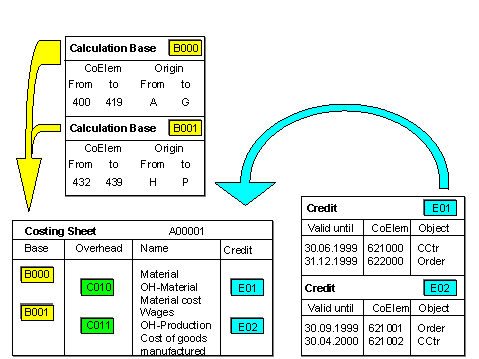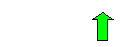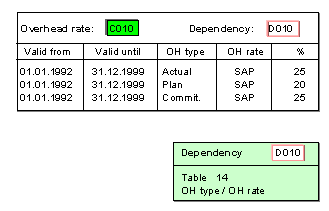• Calculation Base

This defines the primary cost elements to which a particular overhead is to be applied.

This represents a group of cost elements to which you want to apply overhead. For each controlling area, you can assign individual cost elements or cost element intervals and individual origins as well as origin intervals to the calculation bases.

The overhead rate determines to what extent the percentage-based or quantity-based overhead rate should be applied to the direct costs. It also specifies under which conditions (dependencies) the overhead rate is to be applied. For example, you can calculate a defined overhead rate for each plant by specifying a plant dependency.

• Credit

A credit specifies the credit object and the credit cost element.

If an object in actual data is debited with overhead, then another object (such as, a cost center, or order) is credited at the same time. You allocate the overhead using a special overhead cost element (category 41).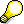Only debits are posted in commitments (no credits).

A costing sheet comprises multiple rows that are processed from top to bottom during the overhead calculation.

• Base Rows

You define these by assigning a calculation base, so that they contain the direct costs that are to have overhead applied to them during the overhead costing.

These are defined by the assignment of an overhead rate.

An overhead row consists of a base row or a totals row. The overhead amount is calculated by multiplying the amount contained in these rows by the overhead percentage rate or quantity-based overhead rate determined through the overhead rates.

As well as overheads, the overhead rows contain credit keys. These credit keys determine which object (such as a cost center or order) is to be credited under which cost element during overhead rate determination.

• Totals Rows

Totals rows are used to generate subtotals or final totals.